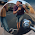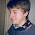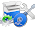## Wednesday, May 1, 2013

### Motivation

It's relatively simple to write a program to approximate derivatives. We simply look at the limit definition of  a derivative:

$$\frac{d}{dx} f(x) = \lim_{h \rightarrow 0} \frac{f(x+h) - f(x)}{h}$$

and choose some small decimal number to use for $h$ instead of  calculating the limit of the function as it goes to $0$. Generally, this works pretty well. We get good approximations as long as $h$ is small enough. However, these approximations are not quite exact, and they can only be evaluated at specific points (for example, we can find $\frac{d}{dx}f(5)$, but not the actual symbolic function). So, what if we want to know what $\frac{d}{dx}f(x)$ is for all x? Calculating derivatives with this approximation method won't do the trick for us -- we'll need something a little more powerful, which is what we will build in this post.

Admittedly, this idea didn't come to me through necessity of such a system; I was just curious. It ended up being pretty enlightening, and it's amazing how simple Haskell makes this type of thing. Most of the code in this post didn't take too long to write, it's relatively short (only 108 lines total, including whitespace) and has a lot of capability.

With that, let's get started!

### The Data Type

I don't want to over-complicate the process of taking derivatives, so my data type for algebraic expressions is kept minimal.

We support six different types of expressions:
• Variables (denoted by a single character)
• Constant values
• Multiplication
• Exponentiation
• Division
This can be expanded upon, but this is adequate for the purpose of demonstration.

Here is our Expr a type, with a sample expression representing $3x^2$:

Haskell allows infix operators to act as data constructors, which allows us to express algebraic expressions cleanly and concisely without too much mental parsing. Also note that, since we explicitly defined operator precedence above the data type declaration, we can write expressions without parentheses according to order of operations, like we did in the sample expression.

### The Algebra

Taking derivatives simply by the rules can get messy, so we might as well go ahead and set up an algebra simplification function that cleans up our final expressions for us. This is actually incredibly simple. As long as you know algebraic laws, this kind of thing basically writes itself in Haskell. We just need to pattern match against certain expressions and meld them together according to algebraic law.

This function ends up being lengthy long due to the fact that symbolic manipulation is mostly just a bunch of different cases, but we can encode algebraic simplification rules for our above data type in a straightforward way.

The following simplify function takes an expression and spits out a simpler one that means the same thing. It's really just a bunch of pattern-matching cases, so feel free to skim it.

I've also included a fullSimplify function that runs simplify on an expression until the current input matches the last output of simplify (which ensures an expression is completely simplified)*. Note that in the simplify function, I've covered a lot of bases, but not all of them. Specifically, division simplification is lacking because it gets complicated quickly and I didn't want to focus on that in this blog post.

We should also note that we don't have a data type expressing subtraction or negative numbers, so we'll deal with that now. In order to express the negation of expressions, we define the negate' function, which basically multiplies expressions by $-1$ and outputs the resultant expression.

Now we have a relatively robust system for computing symbolic expressions. However, we aren't able to actually plug anything into these expressions yet, so we'll fix that now.

*Thanks to zoells on Reddit for the suggestion!

### Evaluating Expressions

The first thing we'll need to do to begin the process of evaluating expressions is write a function to plug in a value for a specific variable. We do this in terms of a function called mapVar, implemented as follows:

mapVar searches through an expression for a specific variable and performs a function on each instance of that variable in the function. plugIn takes a character and a value, and is defined using mapVar to map variables with a specific name to a constant provided by the user.

Now that we have plugIn, we can define two functions: One that takes an expression full of only constants and outputs a result (evalExpr'), and one that will replace a single variable with a constant and output the result (evalExpr):

What we're doing here is simple. With evalExpr', we only need to replace our functional types (:+:, :*:, etc) with the actual functions (+, *, etc). When we run into a Const, we simply replace it with it's inner number value. When we run into a Var, we note that it's not possible to evaluate, and tell the user that there is still a variable in the expression that needs to be plugged in.

With evalExpr, we just plug in a value for a specific variable before evaluating the expression. Simple as that!

Here are some examples of expressions and their evaluations:

*Main> evalExpr 'x' 2 (Var 'x')
2.0
*Main> evalExpr 'a' 3 (Const 3 :+: Var 'a' :*: Const 6)
21.0
*Main> evalExpr 'b' 2 (Var 'b' :/: (Const 2 :*: Var 'b'))
0.5

We can even evaluate multivariate expressions using plugIn:

*Main> evalExpr' . plugIn 'x' 1 $plugIn 'y' 2 (Var 'x' :+: Var 'y') 3.0 Now that we've extended our symbolic expressions to be able to be evaluated, let's do what we set out to do -- find derivatives! ### Derivatives We aren't going to get into super-complicated derivatives involving logorithmic or implicit differentiation, etc. Instead, we'll keep it simple for now, and only adhere to some of the 'simple' derivative rules. We'll need one of them for each of our six expression types: constants, variables, addition, multiplication, division, and exponentiation. We already know the following laws for these from calculus: Differentiation of a constant:$\frac{d}{dx}k = 0$Differentiation of a variable:$\frac{d}{dx}x = 1$Addition differentiation:$\frac{d}{dx}\left(f(x) + g(x)\right) = \frac{d}{dx}f(x) +\frac{d}{dx}g(x)$Power rule (/chain rule):$\frac{d}{dx}f(x)^n = nf(x)^{n-1} \cdot \frac{d}{dx}f(x)$Product rule:$\frac{d}{dx}\left(f(x) \cdot g(x)\right) = \frac{d}{dx}f(x) \cdot g(x) + f(x) \cdot \frac{d}{dx}g(x)$Quotient rule:$\frac{d}{dx}\frac{f(x)}{g(x)} = \frac{\frac{d}{dx}f(x) \cdot g(x) - \frac{d}{dx}g(x) \cdot f(x)}{g(x)^2}$As it turns out, we can almost directly represent this in Haskell. There should be no surprises here -- following along with the above rules, it is relatively easy to see how this function calculates derivatives. We will still error out if we get something like$x^x$as input, as it will require a technique we haven't implemented yet. However, this will suffice for a many different expressions. So, what can we do with this? Well, let's take a look: The first, most obvious thing we'll note when running the derivative function on an expression is that what it produces is rather ugly. To fix this, we'll write a function ddx that will simplify the derivative expression three times to make our output cleaner. (Remember sampleExpr =$3x^2$) *Main> derivative sampleExpr Const 3.0 :*: ((Const 2.0 :*: Var 'x' :^: Const 1.0) :*: Const 1.0) :+: Var 'x' :^: Const 2.0 :*: Const 0.0 *Main> ddx sampleExpr Const 6.0 :*: Var 'x' -- 6x Another thing we can do is get a list of derivatives. The iterate method from the Prelude suits this type of thing perfectly -- we can generate a (infinite!) list of derivatives of a function just by calling iterate ddx. Simple, expressive, and incredibly powerful. *Main> take 3$ ddxs sampleExpr
[Const 3.0 :*: Var 'x' :^: Const 2.0,Const 6.0 :*: Var 'x',Const 6.0] -- [3x^2, 6x, 6]
*Main> let ds = take 4 $ddxs sampleExpr *Main> fmap (evalExpr 'x' 2) ds [12.0,12.0,6.0,0.0] We're also able to grab the$n^{th}$derivative of an expression. We could simply grab the$n^{th}$term of ddxs, but we'll do it without the wasted memory by repeatedly composing ddx$n$times using foldr1 and replicate. *Main> nthDerivative 2 sampleExpr Const 6.0 There's one last thing I want to touch on. Since it's so simple to generate a list of derivatives of a function, why not use that to build functions' Taylor series expansions? ### Taylor Series Expansions The Taylor series expansion of a function$f(x)$about$a$is defined as follows: $$\sum_{n=1}^{\infty} \frac{f^{(n)}(a)}{n!} \cdot (x - a)^n$$ The Maclaurin series expansion for a function is the Taylor series of a function with a = 0, and we will also implement that. Given that we can: • Have multivariate expressions • Easily generate a list of derivatives We can actually find the Taylor series expansion of a function easily. Again, we can almost directly implement this function in Haskell, and evaluating it is no more difficult. To produce a Taylor series, we need a couple of things: • A list of derivatives • A list of indices • A list of factorials We create these three things in where clauses in the taylor declaration. indices are simple, derivs calculates a list of derivatives (using mapVar again to change all variables into$a$s), and facts contains our factorials wrapped in Consts. We generate a list of (expression, index)s in exprs, and then map the "gluing" function series over exprs to produce a list of expressions in the series expansion. We then fmap superSimplify over the list in order to simplify down our expressions, and we get back a list of Taylor series terms for the given expression. The Maclaurin expansion can be defined as mentioned above in terms of the Taylor series, and again, we basically directly encode it (though we do have to re-simplify our expressions due to the plugging in of a variable). Let's take a look at the Taylor expansion for$f(x) = x$. We note that:$f(a) = af'(a) = 1f''(a) = 0$And the rest of the derivatives will be 0. So our Taylor series terms$T_n$should look something like:$T_1 = \frac{a}{1} \cdot (x - a) = a \cdot (x-a)T_2 = \frac{1}{2} \cdot (x-a)^2T_3 = \frac{0}{6} \cdot (x-a)^3 = 0$...and so on. Let's take a look at what taylor produces: *Main> take 3$ taylor (Var 'x')
[Var 'a' :*: (Var 'x' :+: Const (-1.0) :*: Var 'a'),
--^ a * (x-a)
(Const 1.0 :/: Const 2.0) :*: (Var 'x' :+: Const (-1.0) :*: Var 'a') :^: Const 2.0,
--^ 1/2 * (x-a)^2
Const 0.0]

This matches what we determined earlier.

To evaluate a Taylor expression, we need a value for $a$, a value for $x$, and a specified number of terms for precision. We default this precision to 100 terms in the evalTaylor function, and the logic takes place in the evalTaylorWithPrecision function. In this function, we get the Taylor expansion, take the first prec terms, plug in a and x for all values of the function, and finally sum the terms. Maclaurin evaluation is again defined in terms of Taylor evaluation.

Taking a look at the above Taylor series expansion of $f(x) = x$, there is only one term where a zero-valued $a$ will produce any output (namely $\frac{1}{2} \cdot (x-a)^2$). So when we evaluate our Maclaurin series for this function at x, we should simply get back $\frac{1}{2}x^2$. Let's see how it works:

*Main> evalMaclaurin 2 (Var 'x')
2.0 --1/2 2^2 = 1/2 * 4 = 2
*Main> evalMaclaurin 3 (Var 'x')
4.5 -- 1/2 * 3^2 = 1/2 * 9 = 4.5
*Main> evalMaclaurin 10 (Var 'x')
50.0 -- 1/2 * 10^2 = 1/2 * 100 = 50
Looks like everything's working!

Until next time,

Ben

1.You can declare your type an instance of Num, Floating etc. like I did here
https://github.com/hepek/Ramblings/blob/master/symb.lhs

And then you can write your expressions excatly like you would write your programs.

1.Very interesting -- I was actually wondering about that. It's awesome that it works, thanks for sharing!

2.Great article! Nice style.

(you've got a typo in T_1 = a/1 * (x-a) = x*(x-a). (should be a * (x-a)))

1.Thank you, and thanks for letting me know! I'll correct it.

3.Small note: -(a^b) /= (-a)^b

1.Thanks! Someone on Reddit also pointed that out and I've fixed it.

4.You give variables a one-char name, but then ignore the name completeley. You should either just drop the name (so there is only one variable), or treat different variables differently when differentiating. In the latter case, derivative should take a variable as an argument, declaring which variable to differentiate by.

1.Yes, when differentiating I'm expecting a single-variable function, and if it's a multivariate function, the differentiation isn't quite right...

I haven't dealt with differentiation of multivariate functions yet, so I don't exactly know what the process is like, and so I didn't include it.

There's no check on whether or not the functions being differentiated are of a single variable, but I've only used single variable functions.

I initially left out the naming of variables but for the purposes of power series, it's necessary, so I stuck it in. The system's not perfect because of little issues like this as you point out, but it works okay as a demonstration.

Thank you for pointing out the flaw though -- I appreciate the feedback!

5.This comment has been removed by the author.

6.Programming is very interesting and creative thing if you do it with love. Your blog code helps a lot to beginners to learn programming from basic to advance level. I really love this blog because I learn a lot from here and this process is still continuing.
Love from Pro Programmer

7.This comment has been removed by the author.

8.Regardless of whether you are an individual from one the numerous mainstream intuitive polynomial math networks despite everything you have to study and practice a great deal.
algebra